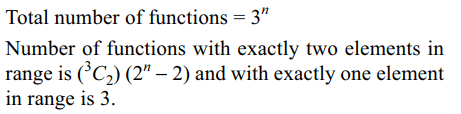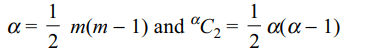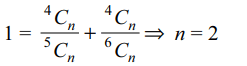## Permutations and Combinations Questions and Answers Part-10

1. The letters of the word SURITI are written in all possible orders and these words are written out as in a dictionary. Then the rank of the word SURITI is
a) 224
b) 245
c) 307
d) 315

Explanation:2. A candidate is required to answer 6 out of 10 questions which are divided into two groups, each containing 5 questions. He is not permitted to attempt more than 4 questions from either group. The number of different ways in which the candidate can choose six questions is
a) 50
b) 150
c) 200
d) 250

Explanation:3. Let A = {1, 2, 3 .... n}, B = {a, b, c}, then the number of functions from A to B that are onto is
a) $3^{n}-3\left(2^{n}-1\right)$
b) $3^{n}-2^{n}$
c) $3\left(2^{n}-1\right)$
d) (n – 1)!

Explanation:4. If $\alpha=^{m}C_{2}$    , where m ≥ 2, then the value of $^{\alpha}C_{2}$  is given by
a) $^{m+1}C_{4}$
b) $^{m-1}C_{4}$
c) $^{m+2}C_{4}$
d) $3\left(^{m+1}C_{4}\right)$

Explanation:5. There are n white and n black balls marked 1, 2, , ...., n. The number of ways in which we can arrange these balls in a row so that neighbouring balls are of different colours is
a) n!
b) (2n)!
c) $2\left(n!\right)^{2}$
d) $\frac{\left(2n\right)!}{\left(n!\right)^{2}}$

Explanation: The balls must be of alternate colours.

6. The number of squares which we can form on a chessboard is
a) 64
b) 160
c) 224
d) 204

Explanation: For a square of size k × k, we have to choose (k + 1) consecutive horizontal and (k + 1) consecutive vertical lines from the chess board, and this can be done in (9 - k) × (9 - k) ways.

7. The number of 5 digit numbers that contain 7 exactly once is
a) $\left(41\right)\left(9^{3}\right)$
b) $\left(37\right)\left(9^{3}\right)$
c) $\left(7\right)\left(9^{4}\right)$
d) $\left(41\right)\left(9^{4}\right)$

Explanation:8. The units digit of $17^{2009}+11^{2009}-7^{2009}$     is
a) 1
b) 8
c) 2
d) 9

Explanation:9. If $\frac{1}{^{4}C_{n}}=\frac{1}{^{5}C_{n}}+\frac{1}{^{6}C_{n}}$    , then value of n is
a) 3
b) 4
c) 1
d) 2d) $2^{5}-2^{3}$